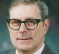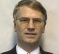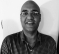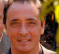## Can you find the illegal step?Started by 2 years ago10 replieslatest reply 2 years ago216 views

Can you find the illegal step in the following silly proof? Given: a=b

$$a^2=ab$$

$$a^2-b^2 = ab-b^2$$

$$(a+b)(a-b)=b(a-b)$$

$$a+b = b$$

$$b+b=b$$

$$2b=b$$

[ - ][ - ]This is mathematics, not real world, isn't it?

The error, if there is one ;-), is the last step where 'b' should be subtracted from both sides, resulting in the true answer 'b=0; => 0=a', !

See also: dy/dx => $inf * 0, doesn't it? (Hence, Are there more infinitesimals than infinities?) Thinking outside the box ;-) [ - ] Reply by August 29, 2021OK OK, the above problem was pretty easy. Can you find the illegal step in the following? Theorem: 4=5 Proof: $$16-36=25-45$$ $$4^2–9*4=5^2–9*5$$ $$4^2–9*4+81/4=5^2–9*5+81/4$$ $$(4-9/2)^2=(5-9/2)^2$$ $$4-9/2=5-9/2$$ $$4=5$$ [ - ] Reply by August 29, 2021Thanks for the mental challenge. It is tricky. The illegal step is taking only one solution from each side when taking the square root. There are two factors on each side of the equation. [ - ] Reply by August 29, 2021If$x^2 = y^2$, taking square root on both sides gives$x = +/- y$. In this case accepting the solution$x = -y$would not have caused a contradiction. Alternatively,$x^2 = y^2$, implies$(x+y)(x-y)=0$, so either$x=y$or$x=-y$or both. Given the specific values of$x$and$y$in this problem, we know that$x=y$is not true, so we must have$x=-y\$.

[ - ]Going from step 3 to step 4, you divided by (a-b). Division by 0 is not meaningful.

[ - ]If you let b = 1, then you get the "classic" homework result:

2 = 1

From this follows the other classic result:

1 = 0.

Neil

[ - ]As a corollary, 2 = 0.

[ - ]